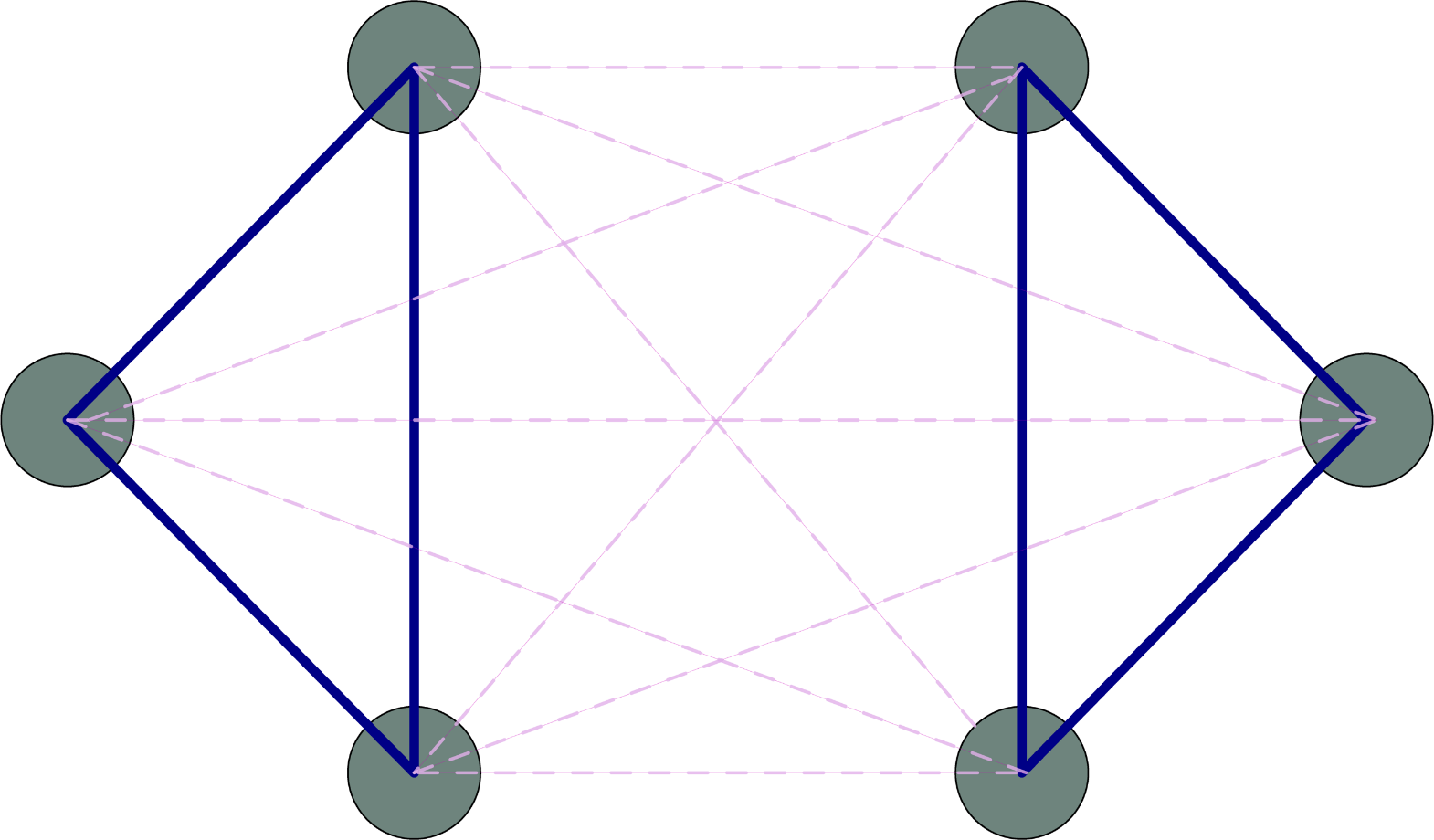# COUPLED OSCILLATORS

### Introduction to Oscillators

An oscillator is anything that moves back and forth, like a pendulum.

Even if something isn’t physically moving back and forth, it can be thought of as an oscillator if it has periodic behavior. For example, fireflies repeatedly illuminate and dim their tails.

It can be useful to think of oscillators as points moving along a circle. To see why, let’s consider a single point moving around a circle at a constant rate.

Notice that the vertical component of the point’s position, shown to the right of the circle, moves back and forth just like a pendulum. This might seem like a cute trick, but it turns out to be a powerful mathematical tool because it lets us use the mathematics of circles to describe oscillations.

### Coupled Oscillators

When mating season arrives on a certain Malaysian riverbank, fireflies gather in great numbers. When night falls they can be seen flashing in unison, a phenomenon known as synchronization

Notice that the vertical component of the point’s position, shown to the right of the circle, moves back and forth just like a pendulum. This might seem like a cute trick, but it turns out to be a powerful mathematical tool because it lets us use the mathematics of circles to describe oscillations.

Somehow, the fireflies are communicating and coordinating their flashes. This is a system of coupled oscillators. We can represent this mathematically as many points on a circle, each rotating at their own natural frequency, but with an added tendency to attract each other.

Even though the points start spread out, after some time they come together to the same point. In other words, the system reaches full synchrony, and the fireflies flash in unison. It turns out there’s a better way to measure synchronization than just watching for the points to clump together. To see how, study the animation below.

The red arrow shown inside the circle is known as the “order parameter”; it points to the average position of all the oscillators. When the system is in a disorganized state the order parameter is in the center of the circle. The more oscillators group together, the larger the order parameter gets, reaching the edge of the circle when all oscillators come together to the same point. Thus, the length of the arrow is a measure of the “level of synchrony” of the system.

### The Two-Population Model and Chimera States

Some animals, like dolphins, sleep with only half of their brain at a time. The brain waves measured from each half look very different. The sleeping half is in a rhythmic state while the alert half is in a more sporadic state, performing many tasks at once. This phenomenon is known as uni-hemispherical sleep. A brain is an extremely complex system: understanding exactly how uni-hemispherical sleep comes about may seem to be a hopelessly daunting task. In order to build up to a full understanding, scientists often begin with simplified models that can be studied more easily. If the simplified model predicts complex behaviors like uni-hemispherical sleep, then we know we’re on the right track!

Neurons fire, relax, and fire again, moving back and forth between two states like an oscillator. This makes systems of coupled oscillators a good starting point to understand some of the brain’s complex behaviors. To mimic a brain’s right and left hemispheres, we can divide the oscillators into two populations, or “hemispheres” as well. The diagram below represents this two-population model. The thick blue lines show the strong connections between neurons of the same hemisphere, and the dotted lines show the weaker connections between neurons of different hemispheres.We can use simulations to try to understand the behavior of this type of system. This next animation shows a simulation of two interacting populations of oscillators connected like the populations in the diagram above. The arrow in each circle is the order parameter for that sub-population.

Take another look at the two populations above. In the left population, all of the oscillators come together to the same point. However, in the right population, the oscillators remain spread out. In other words, the left population is in a fully synchronized state, and the right population is in a partially synchronized state. This can be seen by looking at the order parameters for each population. The left population’s order parameter reaches the edge of the circle, but the right population’s order parameter remains inside.

This is similar to uni-hemispherical sleep. The left population is behaving like the sleeping half of the brain while the right half is awake. What’s particularly remarkable about this situation is that two identical populations end up exhibiting very different behaviors. This type of situation is known as a chimera state. The word “Chimera” comes from Greek mythology, and usually refers to a monstrous fire-breathing hybrid creature composed of parts of more than one animal

### Recap

Let’s recap: we started by describing oscillators: objects that exhibit periodic behavior. We then used oscillators as building blocks in systems of coupled oscillators. These systems exhibited complex behaviors like synchronization and chimera states. This makes systems of coupled oscillators a good simplified model to help understand natural phenomena like synchronized flashing swarms of fireflies and uni-hemispherical sleep.

What other strange or unexpected behaviors can emerge in systems of coupled oscillators? How can we better understand why systems of coupled oscillators exhibit these behaviors? Many scientists and applied mathematicians are hard at work trying to answer these questions!

-